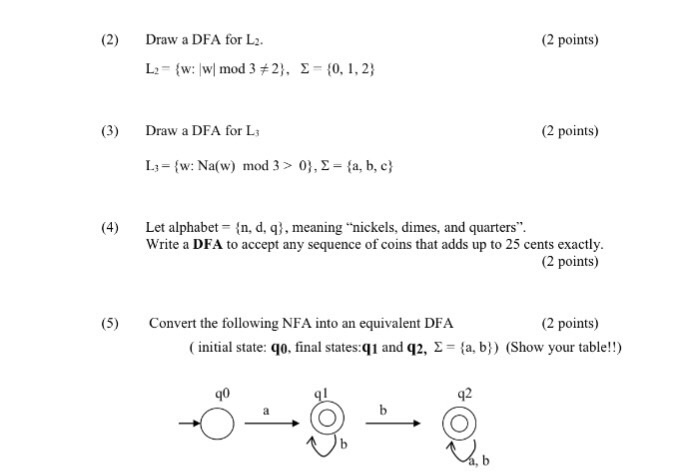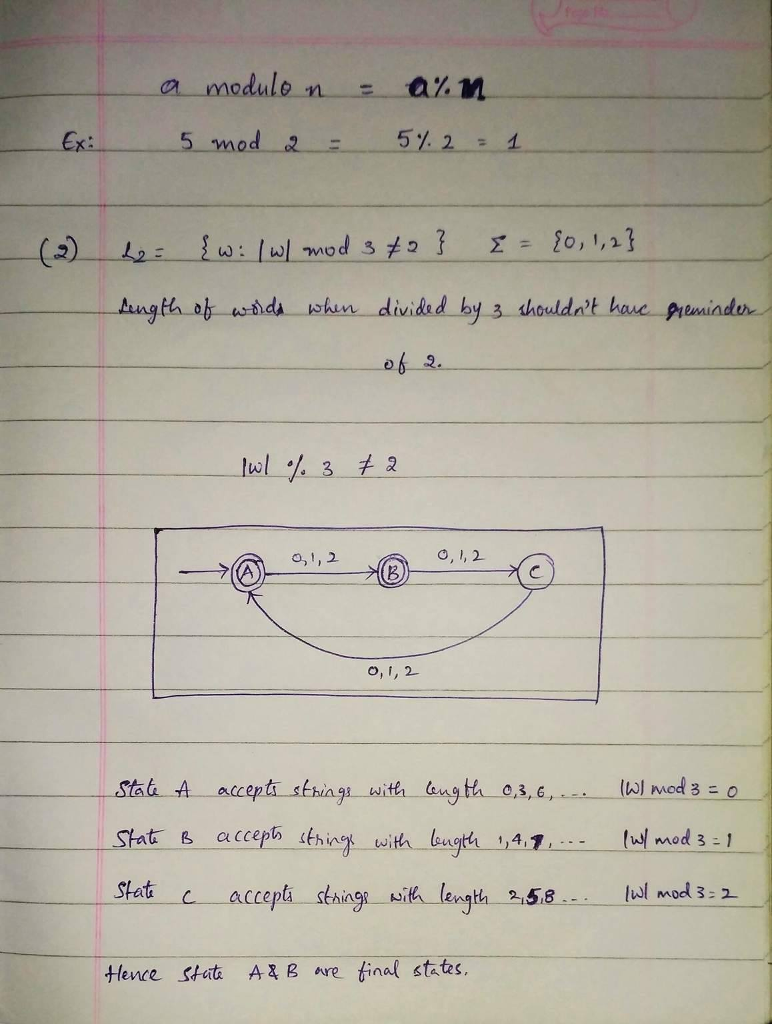# Homework Solution: Draw a DFA for L_2. L_2 = {w: |w| mod 3 notequalto 2}. sigma = {0, 1, 2} Draw a DFA for L_3 L_3 = {w: Na(w) mod 3…Draw a DFA for L_2. L_2 = {w: |w| mod 3 notequalto 2}. sigma = {0, 1, 2} Draw a DFA for L_3 L_3 = {w: Na(w) mod 3 > 0}, sigma = {a, b, c} Let alphabet = {n, d, q} meaning "nickels, dimes, and quarters". Write a DFA to accept any sequence of coins that adds up to 25 cents exactly. Convert the following NFA into an equivalent DFA (initial state: q0, final states: q1 and q2, sigma = {a, b}) (Show your table!!)